# 【深度学习】详解集成学习的投票和Stacking机制1人已赏
Fizz 发布于 2021-06-15 17:25:52 浏览 2892 点赞 93 收藏 5

## 【深度学习】详解集成学习的投票和Stacking机制``````文章目录
1 基础原理
1.1 硬投票
1.2 软投票
2 pytorch综合多个弱分类器，投票机制，进行手写数字分类（boosting）
3 Stacking原理
4 Stacking分类应用
5 kaggle气胸病灶图像分割top4解决方案``````

# 1 基础原理

Hard Voting Classifier（硬投票）：根据少数服从多数来定最终结果； Soft Voting Classifier（软投票）：将所有模型预测样本为某一类别的概率的平均值作为标准，概率最高的对应的类型为最终的预测结果。

## 1.1 硬投票

硬投票通过假设投票最多的类别是获胜者，结合了许多预测。在具有两个类别和三个基础学习器的简单情况下，如果目标类别至少具有两票，则它将成为集成法的最后输出。

模型 1：A - 99%、B - 1%，表示模型 1 认为该样本是 A 类型的概率为 99%，为 B 类型的概率为 1%；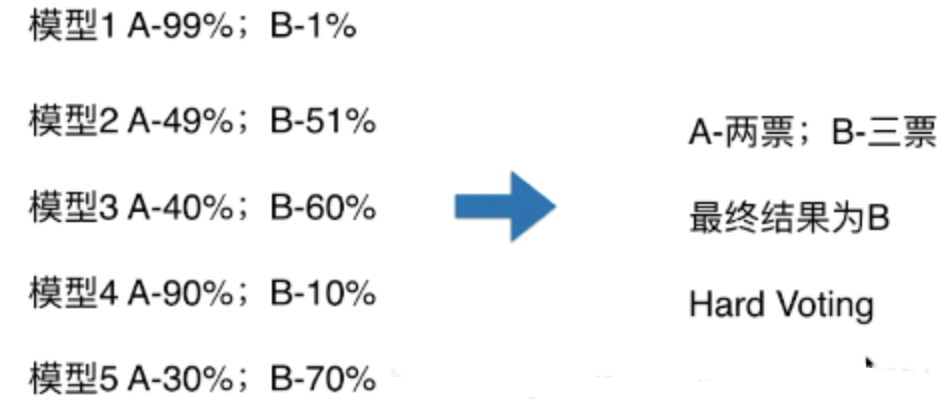## 1.2 软投票

软投票考虑了预测类别的可能性。为了结合预测结果，软投票计算每个类别的平均概率，并假设获胜者是具有最高平均概率的类别。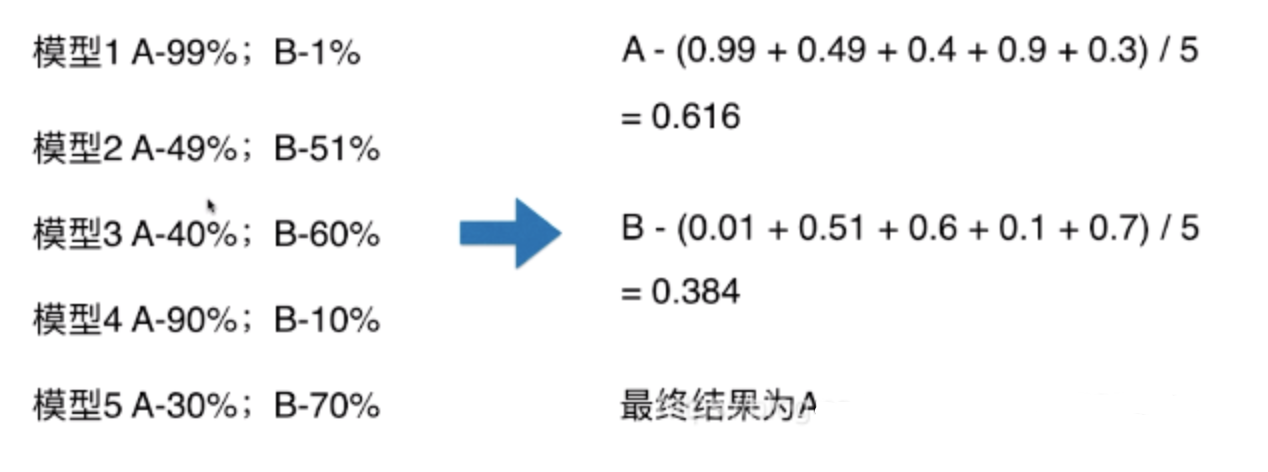如果所有的分类器都能够估计类概率(即sklearn中它们都有一个predict_proba()方法)，那么可以求出类别的概率平均值，投票分类器将具有最高概率的类作为自己的预测。这称为软投票。

在代码中需要两处做更改，在支持向量机中，需要将参数probablity设置为True，使支持向量机具备预测类概率的功能。投票分类器中需要把voting设置为soft 一般soft比hard表现的更出色！

# 2 pytorch综合多个弱分类器，投票机制，进行手写数字分类（boosting）

``````import torch
import torchvision
import torch.nn as nn
from collections import Counter
import numpy as np

class MLP(nn.Module):
def __init__(self):
super(MLP, self).__init__()
self.input_layer=nn.Sequential(
nn.Linear(28*28,30),
nn.Tanh(),
)
self.output_layer=nn.Sequential(
nn.Linear(30,10),
#nn.Sigmoid()
)
def forward(self, x):
x=x.view(x.size(0),-1)
x=self.input_layer(x)
x=self.output_layer(x)
return x

trans=torchvision.transforms.Compose(
[
torchvision.transforms.ToTensor(),
torchvision.transforms.Normalize([.5],[.5]),
]
)
BATCHSIZE=100
EPOCHES=200
LR=0.001

train_data=torchvision.datasets.MNIST(
)
test_data=torchvision.datasets.MNIST(
)

mlps=[MLP().cuda() for i in range(10)]

loss_function=nn.CrossEntropyLoss()

for ep in range(EPOCHES):
img,label=img.cuda(),label.cuda()
for mlp in mlps:
out=mlp(img)
loss=loss_function(out,label)
loss.backward()#网络们获得梯度
optimizer.step()

pre=[]
vote_correct=0
mlps_correct=[0 for i in range(len(mlps))]
img,label=img.cuda(),label.cuda()
for i, mlp in  enumerate( mlps):
out=mlp(img)

_,prediction=torch.max(out,1) #按行取最大值
pre_num=prediction.cpu().numpy()
mlps_correct[i]+=(pre_num==label.cpu().numpy()).sum()

pre.append(pre_num)
arr=np.array(pre)
pre.clear()
result=[Counter(arr[:,i]).most_common(1) for i in range(BATCHSIZE)]
vote_correct+=(result == label.cpu().numpy()).sum()
print("epoch:" + str(ep)+"总的正确率"+str(vote_correct/len(test_data)))

for idx, coreect in enumerate( mlps_correct):
print("网络"+str(idx)+"的正确率为："+str(coreect/len(test_data)))``````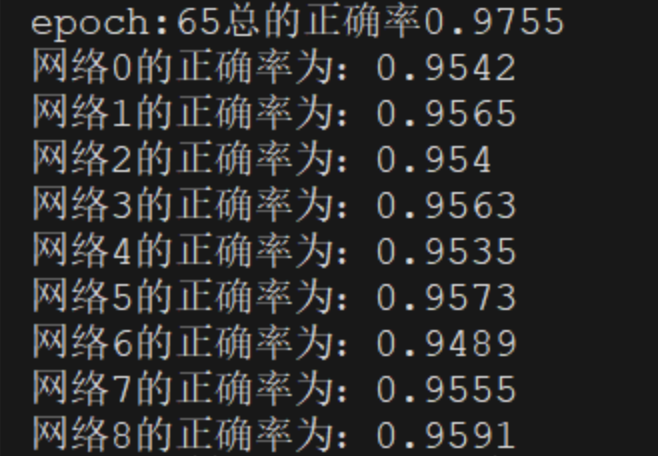可以看到虽然网络模型很简单，但是通过多个弱分类模型的投票，得到的结果也是比其中任何一个网络的效果都要好不少的。应该关注相对提升，不应该关注绝对提升。

# 3 Stacking原理

stacking 就是当用初始训练数据学习出若干个基学习器后，将这几个学习器的预测结果作为新的训练集，来学习一个新的学习器。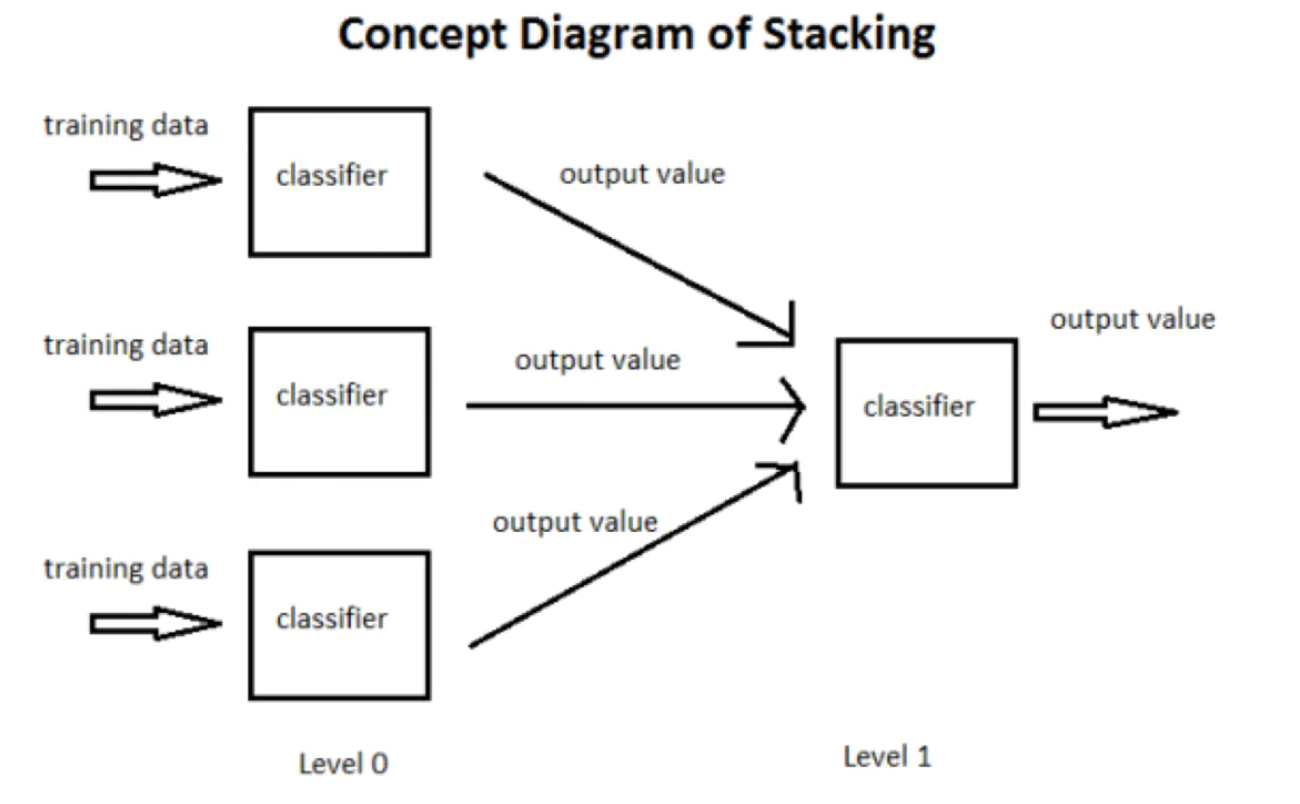他具体是怎么实现的呢，我们来通过代码了解一下吧，代码来源于github https://github.com/log0/vertebral/blob/master/stacked_generalization.py

# 4 Stacking分类应用

clfs = [ RandomForestClassifier(n_estimators = n_trees, criterion = 'gini'), ExtraTreesClassifier(n_estimators = n_trees * 2, criterion = 'gini'), GradientBoostingClassifier(n_estimators = n_trees), ] 接着要训练第一层学习器，并得到第二层学习器所需要的数据，这里会用到 k 折交叉验证。我们首先会将数据集进行一个划分，比如使用80%的训练数据来训练，20%的数据用来测试，

dev_cutoff = len(Y) * 4/5 X_dev = X[:dev_cutoff] Y_dev = Y[:dev_cutoff] X_test = X[dev_cutoff:] Y_test = Y[dev_cutoff:] 然后对训练数据通过交叉验证训练 clf，并得到第二层的训练数据 blend_train,同时，在每个基分类器的每一折交叉验证中，我们都会对测试数据进行一次预测，以得到我们blend_test，二者的定义如下：

blend_train = np.zeros((X_dev.shape, len(clfs))) # Number of training data x Number of classifiers blend_test = np.zeros((X_test.shape, len(clfs))) # Number of testing data x Number of classifiers 按照上面说的，blend_train基于下面的方法得到，注意，下图是对于一个分类器来说的，所以每个分类器得到的blend_train的行数与用于训练的数据一样多，所以blend_train的shape为X_dev.shapelen(clfs)，即训练集长度 基分类器个数：

for j, clf in enumerate(clfs): print 'Training classifier [%s]' % (j) blend_test_j = np.zeros((X_test.shape, len(skf))) # Number of testing data x Number of folds , we will take the mean of the predictions later for i, (train_index, cv_index) in enumerate(skf): print 'Fold [%s]' % (i)

``````        # This is the training and validation set
X_train = X_dev[train_index]
Y_train = Y_dev[train_index]
X_cv = X_dev[cv_index]
Y_cv = Y_dev[cv_index]

clf.fit(X_train, Y_train)

# This output will be the basis for our blended classifier to train against,
# which is also the output of our classifiers
blend_train[cv_index, j] = clf.predict(X_cv)
blend_test_j[:, i] = clf.predict(X_test)
# Take the mean of the predictions of the cross validation set
blend_test[:, j] = blend_test_j.mean(1)``````

bclf = LogisticRegression() bclf.fit(blend_train, Y_dev) 最后，基于我们训练的二级分类器，我们可以预测测试集 blend_test，并得到 score：

Y_test_predict = bclf.predict(blend_test) score = metrics.accuracy_score(Y_test, Y_test_predict) print 'Accuracy = %s' % (score) 如果是多分类怎么办呢，我们这里就不能用predict方法啦，我么要用的是predict_proba方法，得到基分类器对每个类的预测概率代入二级分类器中训练，修改的部分代码如下：

blend_train = np.zeros((np.array(X_dev.values.tolist()).shape, num_classeslen(clfs)),dtype=np.float32) # Number of training data x Number of classifiers blend_test = np.zeros((np.array(X_test.values.tolist()).shape, num_classeslen(clfs)),dtype=np.float32) # Number of testing data x Number of classifiers

``````# For each classifier, we train the number of fold times (=len(skf))
for j, clf in enumerate(clfs):
for i, (train_index, cv_index) in enumerate(skf):
print('Fold [%s]' % (i))

# This is the training and validation set
X_train = X_dev[train_index]
Y_train = Y_dev[train_index]
X_cv = X_dev[cv_index]

X_train = np.concatenate((X_train, ret_x),axis=0)
Y_train = np.concatenate((Y_train, ret_y),axis=0)
clf.fit(X_train, Y_train)
blend_train[cv_index, j*num_classes:(j+1)*num_classes] = clf.predict_proba(X_cv)
blend_test[:, j*num_classes:(j+1)*num_classes] += clf.predict_proba(X_test)``````

blend_test = blend_test / float(n_folds) 上面的代码修改的主要就是blend_train和blend_test的shape，可以看到，对于多分类问题来说，二者的第二维的shape不再是基分类器的数量，而是class的数量*基分类器的数量，这是大家要注意的，否则可能不会得到我们想要的结果。

# 5 kaggle气胸病灶图像分割top4解决方案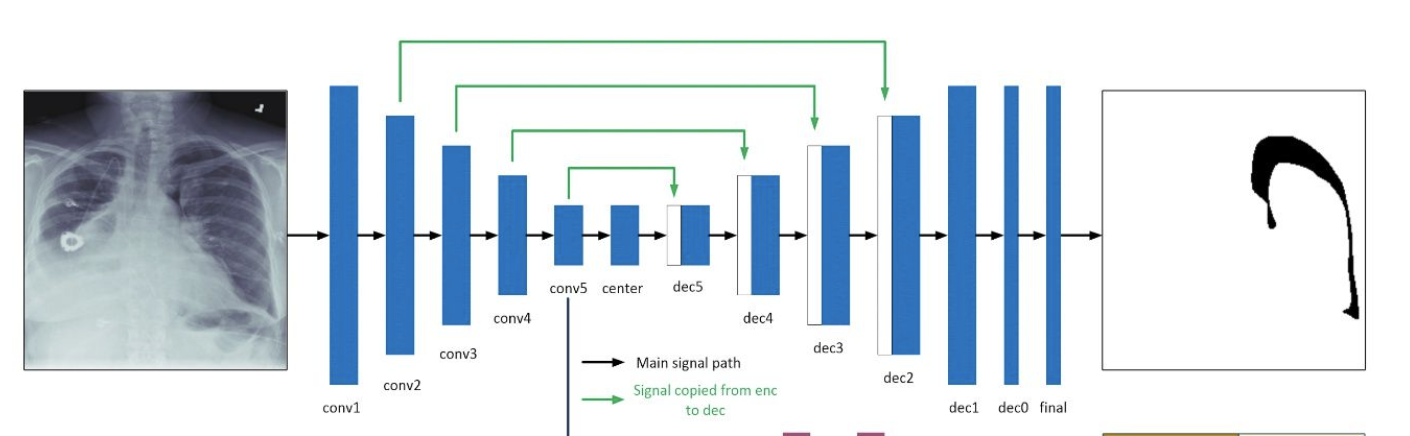模型：Unet 主干网络：ResNet34，固定BN 预处理：在随机裁剪的512x512图片上训练，768x768上进行预测。 数据增强：albumentations中的ShiftScaleRotate, RandomBrightnessContrast, ElasticTransform, HorizontalFlip。 优化器：Adam，批次大小8 Scheduler: CosineAnnealingLR 额外特点：非气胸数据比例在训练过程中逐渐减少，从0.8降至0.22，这样使模型收敛更快。 损失： 2.7 BCE(pred_mask, gt_mask) + 0.9 DICE(pred_mask, gt_mask) + 0.1 * BCE(pred_empty, gt_empty) 后处理： if pred_empty > 0.4 or area(pred_mask) < 800: pred_mask = empty 集成：8折交叉验证得到4个最好模型，使用水平翻转TTA，对结果求平均。

*本文仅代表作者观点，不代表易百纳技术社区立场。系作者授权易百纳技术社区发表，未经许可不得转载。

### 相关专栏海思讨论群君正智能视频群客服微信： x460910098客服手机： 17714209983